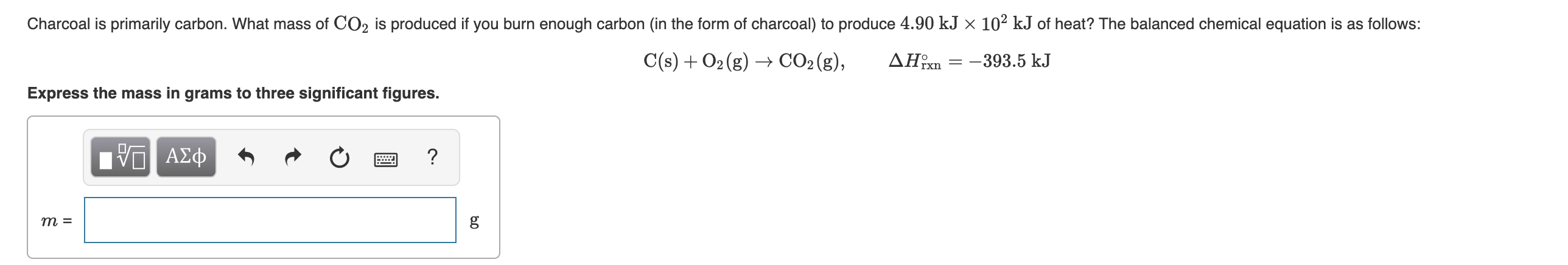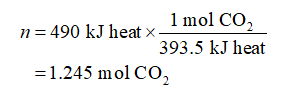# Charcoal is primarily carbon. What mass of CO2 is produced if you burn enough carbon (in the form of charcoal) to produce 4.90 kJ × 10² kJ of heat? The balanced chemical equation is as follows:C(s) + O2 (g) → CO2 (g),ΔΗ-393.5 kJExpress the mass in grams to three significant figures.ο ΑΣφт 3

Question
18 viewshelp_outlineImage TranscriptioncloseCharcoal is primarily carbon. What mass of CO2 is produced if you burn enough carbon (in the form of charcoal) to produce 4.90 kJ × 10² kJ of heat? The balanced chemical equation is as follows: C(s) + O2 (g) → CO2 (g), ΔΗ -393.5 kJ Express the mass in grams to three significant figures. ο ΑΣφ т 3 fullscreen
check_circle

star
star
star
star
star
1 Rating
Step 1

According to the given reaction, 393.5 kJ of heat is produced on the formation of one mole of carbon dioxide (CO2). So, 393.5 kJ of heat is equivalent to 1 mole of CO2. The amount of CO2 to be generated (n) to produce 4.90×102 kJ or 490 kJ of heat is calculated as follows:...

### Want to see the full answer?

See Solution

#### Want to see this answer and more?

Solutions are written by subject experts who are available 24/7. Questions are typically answered within 1 hour.*

See Solution
*Response times may vary by subject and question.
Tagged in

### Chemistry